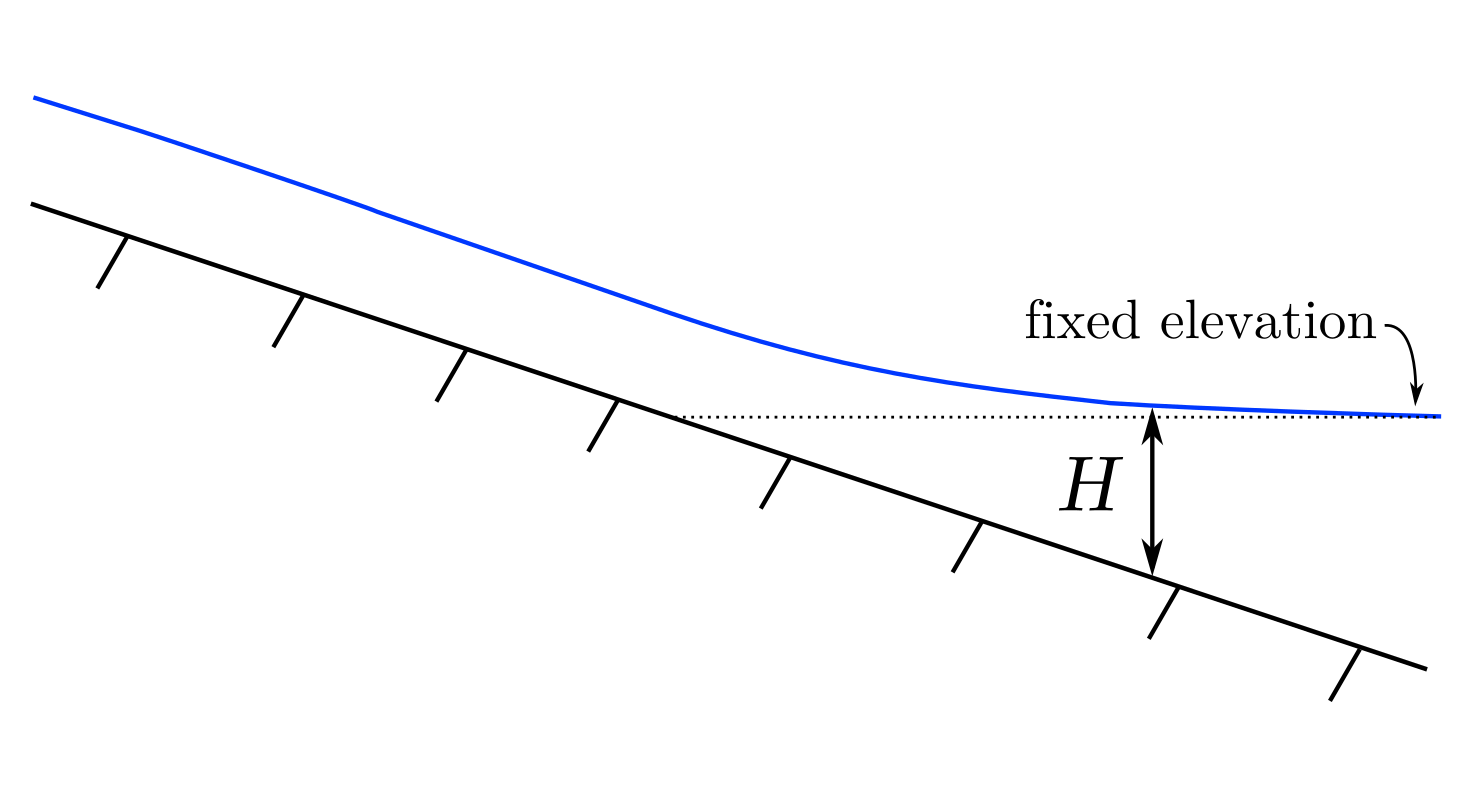# Building a simple delta numerical model: Part I

This is the first in a series I’m going to write over an undetermined-number-of-parts series where we will develop all the pieces to a simple delta numerical model. I’ll outline all the pieces here while I work up an interactive version with a GUI in Matlab, and we will write pieces of the code together within the posts.

The water depth ($H$) over a channel bed with a fixed-elevation downstream boundary can be calculated by a mass-and-momentum conservative derivation following from the full Navier-Stokes equations (e.g., Chow 1959). The so-called backwater equation, in one form, is presented as (Equation 1):

$\frac{dH}{dx} = \frac{S-C_f \textrm{Fr}^2}{(1-\textrm{Fr}^2)}$

where $H$ is the flow depth, $x$ is the streamwise coordinate of the flow, $S$ is the channel bed slope, $C_f$ is the dimensionless coefficient of friction (corresponding to form drag in the channel bed and walls (i.e., roughness and bedforms)), and $\mathrm{Fr}$ is the Froude number, which scales with the ratio of inertia of the flow to the gravitational field driving the flow and can be given as (Equation 2):

$\mathrm{Fr}^2 = \frac{ {q_w} ^2}{gH^3}$

where $q_w = Q_w / B$ is the width-averaged water discharge, and $g$ is the gravitational constant. Equations 1 and 2 are derived for a width-averaged, constant flow width scenario.

Equation 1 is useful to the research we do as scientists of lowland depositional systems because it predicts changes in flow depth through a delta system. The equation is solved with a boundary condition of a known flow depth ($H$) at the fixed-elevation downstream “receiving basin” boundary. For variations in river conditions (i.e., river water discharge), the surface elevation of receiving basins of large lowland rivers are effectively fixed, therefore the equation can be used to estimate a water surface over the lower reaches of the rivers we study for any input discharge conditions!With a calculation of $H$, we can then use a conservation of water-mass equation $Q_w = U A$ (where $U$ is flow velocity and $A = B H$ is channel cross sectional area for an approximately rectangular channel), to evaluate the flow velocity at all points along the channel. Calculation of $U$ in turn allows for calculation of sediment transport, and ultimately sediment deposition—but more on these parts in future parts of this series!

Equation 1 is valid for a solution where width ($B$) is fixed over the downstream length of $x$. Where width is roughly constant, say within the lower reaches of a channel we can use this simple form of the backwater equation. However, where width is not constant over all $x$ (i.e.,$dB/dx \neq 0$), say in the river plume beyond the channel mouth, we must retain an addition term from the derivation from the Navier-Stokes equations to account for this change in width. Since we’re using computers to solve the equations, and the $dB/dx$ term is basically only one extra gradient calculation, this will an easy addition to our model in the future. I’ll write a separate post and link it here with a solution for that version of the backwater equation as well.

So how do we solve Equation 1? Let’s see what we know: the elevation of the receiving basin is fixed at $z = 0$. Therefore, if we know the channel bed profile, we know $H$ at the downstream boundary for ALL possible values of $Qw$. We can evaluate Equation 1 from the known downstream boundary, and knowing all variables on the right hand side at this boundary, calculate the change in flow depth moving upstream over the channel bed ($dH/dx$)!

The solution to Equation 1 (or in its slightly more complex form for a varying width domain) can be numerically estimated pretty easily by a predictor-corrector scheme. We’ll work up a calculation for a fixed-width form of the backwater equation (Equation 1) in the next post, and then proceed to use this as one module of our simple delta model.

This material is based upon work supported by the National Science Foundation (NSF) Graduate Research Fellowship under Grant No. 145068 and NSF EAR-1427177. Any opinion, findings, and conclusions or recommendations expressed in this material are those of the author(s) and do not necessarily reflect the views of the National Science Foundation.

Updated: